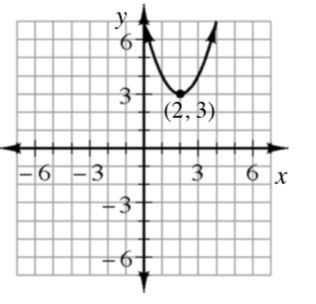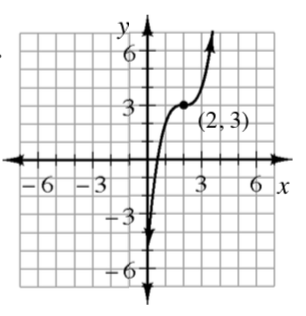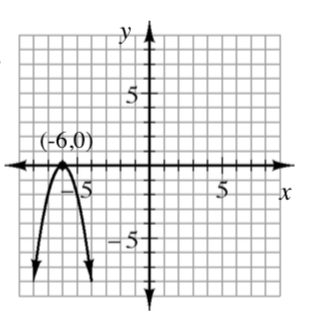### Home > INT3 > Chapter 2 > Lesson 2.2.2 > Problem2-56

2-56.

Use the point $\left(h,k\right)$ to help you write a possible equation for each graph shown below.

1.Remember that $y=(x−h)^2+k$.

Notice that the vertex is $(2,3)$.

$y=(x−2)^2+3$

1.The equation for a cubic graph is $y=(x−h)^3+k$.

$y=(x−2)^3+3$

1.Notice that the parabola opens downs and is steeper.